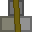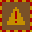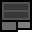# Account 927415

## BalanceMetal: 106s9 = 1705Explosives: 2s0 = 32RC Turrets: 1

## Transfer

 PIN Receiver ReferenceMetal 16 × +Explosives 16 × +RC Turrets

## Create new account token

PIN:

New accounts cost 2 stacks (32 units) metal. You must give the token directly to the other player. Do not create the account yourself and give them the details. If you are found to do this, your account will be closed and all your items taken. You are responsible for ensuring the player you provide a new account token to knows how to use dredbank properly. If many players are found who don't know how to use dredbank properly, and they all got their accounts from one player, there may be a punishment against the player who provided their accounts.

## Transactions

Type Counterparty Account Reference NumberMetalExplosivesRC Turrets
Deposit 0 48s0 = 768 0s0 = 0 0
Sent 837562 0 5s0 = 80 0s0 = 0 0
Received 874245 0 40s0 = 640 0s0 = 0 0
Sent 837562 0 5s0 = 80 0s0 = 0 0
Sent 874245 0 45s0 = 720 0s0 = 0 0
Deposit 0 50s15 = 815 0s0 = 0 0
Received 837562 0 15s0 = 240 0s0 = 0 1
Received 837562 0 3s11 = 59 0s0 = 0 0
Sent 284644 0 5s0 = 80 0s0 = 0 0
Received 984526 0 0s0 = 0 2s0 = 32 0
Sent 874245 0 3s0 = 48 0s0 = 0 0
Sent 837562 0 0s0 = 0 11s0 = 176 0
Received 837562 0 11s0 = 176 0s0 = 0 0
Deposit 0 0s0 = 0 11s0 = 176 0
Sent 653090 0 0s1 = 1 0s0 = 0 0
Deposit 0 1s0 = 16 0s0 = 0 0